Oracle® CEP CQL Language Reference 11g Release 1 (11.1.1) E12048-02

9 Functions: java.lang.Math

Oracle CQL provides a variety of built-in functions based on the java.lang.Math class.

9.1 Introduction to Oracle CQL Built-In java.lang.Math Functions

Table 9-1 lists the built-in java.lang.Math functions that Oracle CQL provides.

Table 9-1 Oracle CQL Built-in java.lang.Math Functions

Type Function

Trigonometric

Logarithmic

Euler's Number

Roots

Signum Function

Unit of Least Precision

Other

 Note: Built-in function names are case sensitive and you must use them in the case shown (in lower case).

 Note: In stream input examples, lines beginning with h (such as h 3800) are heartbeat input tuples. These inform Oracle CEP that no further input will have a timestamp lesser than the heartbeat value.

abs

Syntax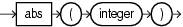Purpose

abs returns the absolute value of the input integer argument as an integer.

Examples

Consider the query q66 in Example 9-1. Given the data stream SFunc with schema (c1 integer, c2 double, c3 bigint) in Example 9-2, the query returns the stream in Example 9-3.

Example 9-1 abs Function Query

<query id="q66"><![CDATA[
select abs(c1) from SFunc
]]></query>

Example 9-2 abs Function Stream Input

Timestamp   Tuple
10         1,0.5,8
1000        -4,0.7,6
1200        -3,0.89,12
2000         8,0.4,4

Example 9-3 abs Function Stream Output

Timestamp   Tuple Kind  Tuple
10:       +           1
1000:       +           4
1200:       +           3
2000:       +           8

abs1

Syntax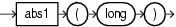Purpose

abs1 returns the absolute value of the input long argument as a long.

Examples

Consider the query q67 in Example 9-4. Given the data stream SFunc with schema (c1 integer, c2 float, c3 long) in Example 9-5, the query returns the stream in Example 9-6.

Example 9-4 abs1 Function Query

<query id="q67"><![CDATA[
select abs1(c3) from SFunc
]]></query>

Example 9-5 abs1 Function Stream Input

Timestamp   Tuple
10        1,0.5,8
1000        4,0.7,-6
1200        3,0.89,-12
2000        8,0.4,4

Example 9-6 abs1 Function Stream Output

Timestamp   Tuple Kind  Tuple
10:       +           8
1000:       +           6
1200:       +           12
2000:       +           4

abs2

Syntax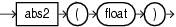Purpose

abs2 returns the absolute value of the input float argument as a float.

Examples

Consider the query q68 in Example 9-7. Given the data stream SFunc with schema (c1 integer, c2 float, c3 bigint) in Example 9-8, the query returns the stream in Example 9-9.

Example 9-7 abs2 Function Query

<query id="q68"><![CDATA[
select abs2(c2) from SFunc
]]></query>

Example 9-8 abs2 Function Stream Input

Timestamp   Tuple
10        1,0.5,8
1000        4,-0.7,6
1200        3,-0.89,12
2000        8,0.4,4

Example 9-9 abs2 Function Stream Output

Timestamp   Tuple Kind  Tuple
10:       +           0.5
1000:       +           0.7
1200:       +           0.89
2000:       +           0.4

abs3

Syntax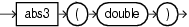Purpose

abs3 returns the absolute value of the input double argument as a double.

Examples

Consider the query q69 in Example 9-10. Given the data stream SFunc with schema (c1 integer, c2 float, c3 bigint, c4 double) in Example 9-11, the query returns the stream in Example 9-12.

Example 9-10 abs3 Function Query

<query id="q69"><![CDATA[
select abs3(c4) from SFunc
]]></query>

Example 9-11 abs3 Function Stream Input

Timestamp   Tuple
10        1,0.5,8,0.25334
1000        4,0.7,6,-4.64322
1200        3,0.89,12,-1.4672272
2000        8,0.4,4,2.66777

Example 9-12 abs3 Function Stream Output

Timestamp   Tuple Kind  Tuple
10:       +           0.25334
1000:       +           4.64322
1200:       +           1.4672272
2000:       +           2.66777

acos

Syntax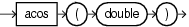Purpose

acos returns the arc cosine of a double angle, in the range of 0.0 through pi, as a double.

Examples

Consider the query q73 in Example 9-13. Given the data stream SFunc with schema (c1 integer, c2 double, c3 bigint) in Example 9-14, the query returns the stream in Example 9-15.

Example 9-13 acos Function Query

<query id="q73"><![CDATA[
select acos(c2) from SFunc
]]></query>

Example 9-14 acos Function Stream Input

Timestamp   Tuple
10        1,0.5,8
1000        4,0.7,6
1200        3,0.89,12
2000        8,0.4,4

Example 9-15 acos Function Stream Output

Timestamp   Tuple Kind  Tuple
10:       +           1.0471976
1000:       +           0.79539883
1200:       +           0.4734512
2000:       +           1.1592795

asin

Syntax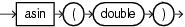Purpose

asin returns the arc sine of a double angle, in the range of -pi/2 through pi/2, as a double.

Examples

Consider the query q74 in Example 9-16. Given the data stream SFunc with schema (c1 integer, c2 double, c3 bigint) in Example 9-17, the query returns the stream in Example 9-18.

Example 9-16 asin Function Query

<query id="q74"><![CDATA[
select asin(c2) from SFunc
]]></query>

Example 9-17 asin Function Stream Input

Timestamp   Tuple
10        1,0.5,8
1000        4,0.7,6
1200        3,0.89,12
2000        8,0.4,4

Example 9-18 asin Function Stream Output

Timestamp   Tuple Kind  Tuple
10:       +           0.5235988
1000:       +           0.7753975
1200:       +           1.0973451
2000:       +           0.41151685

atan

Syntax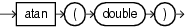Purpose

atan returns the arc tangent of a double angle, in the range of -pi/2 through pi/2, as a double.

Examples

Consider the query q75 in Example 9-19. Given the data stream SFunc with schema (c1 integer, c2 double, c3 bigint) in Example 9-20, the query returns the stream in Example 9-21.

Example 9-19 atan Function Query

<query id="q75"><![CDATA[
select atan(c2) from SFunc
]]></query>

Example 9-20 atan Function Stream Input

Timestamp   Tuple
10        1,0.5,8
1000        4,0.7,6
1200        3,0.89,12
2000        8,0.4,4

Example 9-21 atan Function Stream Output

Timestamp   Tuple Kind  Tuple
10:       +           0.4636476
1000:       +           0.61072594
1200:       +           0.7272627
2000:       +           0.3805064

atan2

Syntax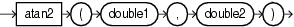Purpose

atan2 converts rectangular coordinates (x,y) to polar (r,theta) coordinates.

This function takes the following arguments:

• double1: the ordinate coordinate.

• double2: the abscissa coordinate.

This function returns the theta component of the point (r,theta) in polar coordinates that corresponds to the point (x,y) in Cartesian coordinates as a double.

Examples

Consider the query q63 in Example 9-22. Given the data stream SFunc with schema (c1 integer, c2 double, c3 bigint) in Example 9-23, the query returns the stream in Example 9-24.

Example 9-22 atan2 Function Query

<query id="q63"><![CDATA[
select atan2(c2,c2) from SFunc
]]></query>

Example 9-23 atan2 Function Stream Input

Timestamp   Tuple
10        1,0.5,8
1000        4,0.7,6
1200        3,0.89,12
2000        8,0.4,4

Example 9-24 atan2 Function Stream Output

Timestamp   Tuple Kind  Tuple
10:       +           0.7853982
1000:       +           0.7853982
1200:       +           0.7853982
2000:       +           0.7853982

cbrt

Syntax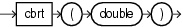Purpose

cbrt returns the cube root of the double argument as a double.

For positive finite a, cbrt(-a) == -cbrt(a); that is, the cube root of a negative value is the negative of the cube root of that value's magnitude.

Examples

Consider the query q76 in Example 9-25. Given the data stream SFunc with schema (c1 integer, c2 float, c3 bigint) in Example 9-26, the query returns the stream in Example 9-27.

Example 9-25 cbrt Function Query

<query id="q76"><![CDATA[
select cbrt(c2) from SFunc
]]></query>

Example 9-26 cbrt Function Stream Input

Timestamp   Tuple
10        1,0.5,8
1000        4,0.7,6
1200        3,0.89,12
2000        8,0.4,4

Example 9-27 cbrt Function Stream Output

Timestamp   Tuple Kind  Tuple
10:       +           0.7937005
1000:       +           0.887904
1200:       +           0.9619002
2000:       +           0.73680633

ceil1

Syntax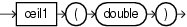Purpose

ceil1 returns the smallest (closest to negative infinity) double value that is greater than or equal to the double argument and equals a mathematical integer.

To avoid possible rounding error, consider using (long) cern.jet.math.Arithmetic.ceil(double).

Examples

Consider the query q77 in Example 9-28. Given the data stream SFunc with schema (c1 integer, c2 double, c3 bigint) in Example 9-29, the query returns the stream in Example 9-30.

Example 9-28 ceil1 Function Query

<query id="q77"><![CDATA[
select ceil1(c2) from SFunc
]]></query>

Example 9-29 ceil1 Function Stream Input

Timestamp   Tuple
10        1,0.5,8
1000        4,0.7,6
1200        3,0.89,12
2000        8,0.4,4

Example 9-30 ceil1 Function Stream Output

Timestamp   Tuple Kind  Tuple
10:       +           1.0
1000:       +           1.0
1200:       +           1.0
2000:       +           1.0

cos

Syntax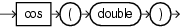Purpose

cos returns the trigonometric cosine of a double angle as a double.

Examples

Consider the query q61 in Example 9-31. Given the data stream SFunc with schema (c1 integer, c2 double, c3 bigint) in Example 9-32, the query returns the stream in Example 9-33.

Example 9-31 cos Function Query

<query id="q61"><![CDATA[
select cos(c2) from SFunc
]]></query>

Example 9-32 cos Function Stream Input

Timestamp   Tuple
10        1,0.5,8
1000        4,0.7,6
1200        3,0.89,12
2000        8,0.4,4

Example 9-33 cos Function Stream Output

Timestamp   Tuple Kind  Tuple
10:       +           0.87758255
1000:       +           0.7648422
1200:       +           0.62941206
2000:       +           0.921061

cosh

Syntax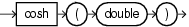Purpose

cosh returns the hyperbolic cosine of a double value as a double.

Examples

Consider the query q78 in Example 9-34. Given the data stream SFunc with schema (c1 integer, c2 double, c3 bigint) in Example 9-35, the query returns the stream in Example 9-36.

Example 9-34 cosh Function Query

<query id="q78"><![CDATA[
select cosh(c2) from SFunc
]]></query>

Example 9-35 cosh Function Stream Input

Timestamp   Tuple
10        1,0.5,8
1000        4,0.7,6
1200        3,0.89,12
2000        8,0.4,4

Example 9-36 cosh Function Stream Output

Timestamp   Tuple Kind  Tuple
10:       +           1.127626
1000:       +           1.255169
1200:       +           1.4228927
2000:       +           1.0810723

exp

Syntax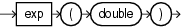Purpose

exp returns Euler's number e raised to the power of the double argument as a double.

Note that for values of x near 0, the exact sum of expm1(x) + 1 is much closer to the true result of Euler's number e raised to the power of x than EXP(x).

Examples

Consider the query q79 in Example 9-37. Given the data stream SFunc with schema (c1 integer, c2 double, c3 bigint) in Example 9-38, the query returns the stream in Example 9-39.

Example 9-37 exp Function Query

<query id="q79"><![CDATA[
select exp(c2) from SFunc
]]></query>

Example 9-38 exp Function Stream Input

Timestamp   Tuple
10        1,0.5,8
1000        4,0.7,6
1200        3,0.89,12
2000        8,0.4,4

Example 9-39 exp Function Stream Output

Timestamp   Tuple Kind  Tuple
10:       +           1.6487212
1000:       +           2.0137527
1200:       +           2.4351296
2000:       +           1.4918247

expm1

Syntax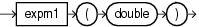Purpose

expm1 returns the computation that Figure 9-1 shows as a double, where x is the double argument and e is Euler's number.

Figure 9-1 java.lang.Math Expm1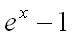Note that for values of x near 0, the exact sum of expm1(x) + 1 is much closer to the true result of Euler's number e raised to the power of x than exp(x).

Examples

Consider the query q80 in Example 9-40. Given the data stream SFunc with schema (c1 integer, c2 double, c3 bigint) in Example 9-41, the query returns the stream in Example 9-42.

Example 9-40 expm1 Function Query

<query id="q80"><![CDATA[
select expm1(c2) from SFunc
]]></query>

Example 9-41 expm1 Function Stream Input

Timestamp   Tuple
10        1,0.5,8
1000        4,0.7,6
1200        3,0.89,12
2000        8,0.4,4

Example 9-42 expm1 Function Stream Output

Timestamp   Tuple Kind  Tuple
10:       +           0.6487213
1000:       +           1.0137527
1200:       +           1.4351296
2000:       +           0.49182472

floor1

Syntax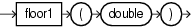Purpose

floor1 returns the largest (closest to positive infinity) double value that is less than or equal to the double argument and equals a mathematical integer.

To avoid possible rounding error, consider using (long) cern.jet.math.Arithmetic.floor(double).

Examples

Consider the query q81 in Example 9-43. Given the data stream SFunc with schema (c1 integer, c2 double, c3 bigint) in Example 9-44, the query returns the stream in Example 9-45.

Example 9-43 floor1 Function Query

<query id="q81"><![CDATA[
select floor1(c2) from SFunc
]]></query>

Example 9-44 floor1 Function Stream Input

Timestamp   Tuple
10        1,0.5,8
1000        4,0.7,6
1200        3,0.89,12
2000        8,0.4,4

Example 9-45 floor1 Function Stream Output

Timestamp   Tuple Kind  Tuple
10:       +           0.0
1000:       +           0.0
1200:       +           0.0
2000:       +           0.0

hypot

Syntax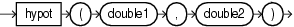Purpose

hypot returns the hypotenuse (see Figure 9-2) of the double arguments as a double.

Figure 9-2 java.lang.Math hypot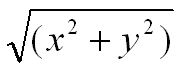This function takes the following arguments:

• double1: the x value.

• double2: the y value.

The hypotenuse is computed without intermediate overflow or underflow.

Examples

Consider the query q82 in Example 9-46. Given the data stream SFunc with schema (c1 integer, c2 double, c3 bigint) in Example 9-47, the query returns the stream in Example 9-48.

Example 9-46 hypot Function Query

<query id="q82"><![CDATA[
select hypot(c2,c2) from SFunc
]]></query>

Example 9-47 hypot Function Stream Input

Timestamp   Tuple
10        1,0.5,8
1000        4,0.7,6
1200        3,0.89,12
2000        8,0.4,4

Example 9-48 hypot Function Stream Output

Timestamp   Tuple Kind  Tuple
10:       +           0.70710677
1000:       +           0.98994946
1200:       +           1.2586501
2000:       +           0.56568545

ieeeremainder

Syntax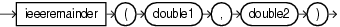Purpose

ieeeremainder computes the remainder operation on two double arguments as prescribed by the IEEE 754 standard and returns the result as a double.

This function takes the following arguments:

• double1: the dividend.

• double2: the divisor.

The remainder value is mathematically equal to f1 - f2 × n, where n is the mathematical integer closest to the exact mathematical value of the quotient f1/f2, and if two mathematical integers are equally close to f1/f2, then n is the integer that is even. If the remainder is zero, its sign is the same as the sign of the first argument.

Examples

Consider the query q72 in Example 9-49. Given the data stream SFunc with schema (c1 integer, c2 double, c3 bigint) in Example 9-50, the query returns the stream in Example 9-51.

Example 9-49 ieeeremainder Function Query

<query id="q72"><![CDATA[
select ieeeremainder(c2,c2) from SFunc
]]></query>

Example 9-50 ieeeremainder Function Stream Input

Timestamp   Tuple
10        1,0.5,8
1000        4,0.7,6
1200        3,0.89,12
2000        8,0.4,4

Example 9-51 ieeeremainder Function Stream Output

Timestamp   Tuple Kind  Tuple
10:       +           0.0
1000:       +           0.0
1200:       +           0.0
2000:       +           0.0

log1

Syntax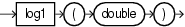Purpose

log1 returns the natural logarithm (base e) of a double value as a double.

Note that for small values x, the result of log1p(x) is much closer to the true result of ln(1 + x) than the floating-point evaluation of log(1.0+x).

Examples

Consider the query q83 in Example 9-52. Given the data stream SFunc with schema (c1 integer, c2 double, c3 bigint) in Example 9-53, the query returns the stream in Example 9-54.

Example 9-52 log1 Function Query

<query id="q83"><![CDATA[
select log1(c2) from SFunc
]]></query>

Example 9-53 log1 Function Stream Input

Timestamp   Tuple
10        1,0.5,8
1000        4,0.7,6
1200        3,0.89,12
2000        8,0.4,4

Example 9-54 log1 Function Stream Output

Timestamp   Tuple Kind  Tuple
10:       +           -0.6931472
1000:       +           -0.35667497
1200:       +           -0.11653383
2000:       +           -0.9162907

log101

SyntaxPurpose

log101 returns the base 10 logarithm of a double value as a double.

Examples

Consider the query q84 in Example 9-55. Given the data stream SFunc with schema (c1 integer, c2 double, c3 bigint) in Example 9-56, the query returns the stream in Example 9-57.

Example 9-55 log101 Function Query

<query id="q84"><![CDATA[
select log101(c2) from SFunc
]]></query>

Example 9-56 log101 Function Stream Input

Timestamp   Tuple
10        1,0.5,8
1000        4,0.7,6
1200        3,0.89,12
2000        8,0.4,4

Example 9-57 log101 Function Stream Output

Timestamp   Tuple Kind  Tuple
10:       +           -0.30103
1000:       +           -0.15490197
1200:       +           -0.050610002
2000:       +           -0.39794

log1p

Syntax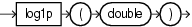Purpose

log1p returns the natural logarithm of the sum of the double argument and 1 as a double.

Note that for small values x, the result of log1p(x) is much closer to the true result of ln(1 + x) than the floating-point evaluation of log(1.0+x).

Examples

Consider the query q85 in Example 9-58. Given the data stream SFunc with schema (c1 integer, c2 double, c3 bigint) in Example 9-59, the query returns the stream in Example 9-60.

Example 9-58 log1p Function Query

<query id="q85"><![CDATA[
select log1p(c2) from SFunc
]]></query>

Example 9-59 log1p Function Stream Input

Timestamp   Tuple
10        1,0.5,8
1000        4,0.7,6
1200        3,0.89,12
2000        8,0.4,4

Example 9-60 log1p Function Stream Output

Timestamp   Tuple Kind  Tuple
10:       +           0.4054651
1000:       +           0.53062826
1200:       +           0.63657683
2000:       +           0.33647224

pow

Syntax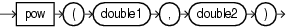Purpose

pow returns the value of the first double argument (the base) raised to the power of the second double argument (the exponent) as a double.

This function takes the following arguments:

• double1: the base.

• double2: the exponent.

Examples

Consider the query q65 in Example 9-61. Given the data stream SFunc with schema (c1 integer, c2 double, c3 bigint) in Example 9-62, the query returns the stream in Example 9-63.

Example 9-61 pow Function Query

<query id="q65"><![CDATA[
select pow(c2,c2) from SFunc
]]></query>

Example 9-62 pow Function Stream Input

Timestamp   Tuple
10        1,0.5,8
1000        4,0.7,6
1200        3,0.89,12
2000        8,0.4,4

Example 9-63 pow Function Stream Output

Timestamp   Tuple Kind  Tuple
10:       +           0.70710677
1000:       +           0.7790559
1200:       +           0.9014821
2000:       +           0.69314486

rint

Syntax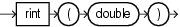Purpose

rint returns the double value that is closest in value to the double argument and equals a mathematical integer. If two double values that are mathematical integers are equally close, the result is the integer value that is even.

Examples

Consider the query q86 in Example 9-64. Given the data stream SFunc with schema (c1 integer, c2 double, c3 bigint) in Example 9-65, the query returns the stream in Example 9-66.

Example 9-64 rint Function Query

<query id="q86"><![CDATA[
select rint(c2) from SFunc
]]></query>

Example 9-65 rint Function Stream Input

Timestamp   Tuple
10        1,0.5,8
1000        4,0.7,6
1200        3,0.89,12
2000        8,0.4,4

Example 9-66 rint Function Stream Output

Timestamp   Tuple Kind  Tuple
10:       +           0.0
1000:       +           1.0
1200:       +           1.0
2000:       +           0.0

round

Syntax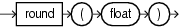Purpose

round returns the closest integer to the float argument.

Examples

Consider the query q87 in Example 9-67. Given the data stream SFunc with schema (c1 integer, c2 double, c3 bigint) in Example 9-68, the query returns the stream in Example 9-69.

Example 9-67 round Function Query

<query id="q87"><![CDATA[
select round(c2) from SFunc
]]></query>

Example 9-68 round Function Stream Input

Timestamp   Tuple
10        1,0.5,8
1000        4,0.7,6
1200        3,0.89,12
2000        8,0.4,4

Example 9-69 round Function Stream Output

Timestamp   Tuple Kind  Tuple
10:       +           1
1000:       +           1
1200:       +           1
2000:       +           0

round1

Syntax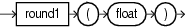Purpose

round1 returns the closest integer to the float argument.

Examples

Consider the query q88 in Example 9-70. Given the data stream SFunc with schema (c1 integer, c2 double, c3 bigint) in Example 9-71, the query returns the stream in Example 9-72.

Example 9-70 round1 Function Query

<query id="q88"><![CDATA[
select round1(c2) from SFunc
]]></query>

Example 9-71 round1 Function Stream Input

Timestamp   Tuple
10        1,0.5,8
1000        4,0.7,6
1200        3,0.89,12
2000        8,0.4,4

Example 9-72 round1 Function Stream Output

Timestamp   Tuple Kind  Tuple
10:       +           1
1000:       +           1
1200:       +           1
2000:       +           0

signum

Syntax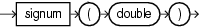Purpose

signum returns the signum function of the double argument as a double:

• zero if the argument is zero

• 1.0 if the argument is greater than zero

• -1.0 if the argument is less than zero

Examples

Consider the query q70 in Example 9-73. Given the data stream SFunc with schema (c1 integer, c2 double, c3 bigint) in Example 9-74, the query returns the stream in Example 9-75.

Example 9-73 signum Function Query

<query id="q70"><![CDATA[
select signum(c2) from SFunc
]]></query>

Example 9-74 signum Function Stream Input

Timestamp   Tuple
10        1,0.5,8
1000        4,-0.7,6
1200        3,-0.89,12
2000        8,0.4,4

Example 9-75 signum Function Stream Output

Timestamp   Tuple Kind  Tuple
10:       +           1.0
1000:       +           -1.0
1200:       +           -1.0
2000:       +           1.0

signum1

Syntax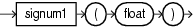Purpose

signum1 returns the signum function of the float argument as a float:

• zero if the argument is zero

• 1.0 if the argument is greater than zero

• -1.0 if the argument is less than zero

Examples

Consider the query q71 in Example 9-76. Given the data stream SFunc with schema (c1 integer, c2 double, c3 bigint) in Example 9-77, the query returns the relation in Example 9-78.

Example 9-76 signum1 Function Query

<query id="q71"><![CDATA[
select signum1(c2) from SFunc
]]></query>

Example 9-77 signum1 Function Stream Input

Timestamp   Tuple
10        1,0.5,8
1000        4,-0.7,6
1200        3,-0.89,12
2000        8,0.4,4

Example 9-78 signum1 Function Relation Output

Timestamp   Tuple Kind  Tuple
10:       +           1.0
1000:       +           -1.0
1200:       +           -1.0
2000:       +           1.0

sin

Syntax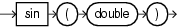Purpose

sin returns the trigonometric sine of a double angle as a double.

Examples

Consider the query q60 in Example 9-79. Given the data stream SFunc with schema (c1 integer, c2 float, c3 bigint) in Example 9-80, the query returns the stream in Example 9-81.

Example 9-79 sin Function Query

<query id="q60"><![CDATA[
select sin(c2) from SFunc
]]></query>

Example 9-80 sin Function Stream Input

Timestamp   Tuple
10        1,0.5,8
1000        4,0.7,6
1200        3,0.89,12
2000        8,0.4,4

Example 9-81 sin Function Stream Output

Timestamp   Tuple Kind  Tuple
10:       +           0.47942555
1000:       +           0.64421767
1200:       +           0.7770717
2000:       +           0.38941833

sinh

Syntax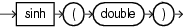Purpose

sinh returns the hyperbolic sine of a double value as a double.

Examples

Consider the query q89 in Example 9-82. Given the data stream SFunc with schema (c1 integer, c2 double, c3 bigint) in Example 9-83, the query returns the stream in Example 9-84.

Example 9-82 sinh Function Query

<query id="q89"><![CDATA[
select sinh(c2) from SFunc
]]></query>

Example 9-83 sinh Function Stream Input

Timestamp   Tuple
10        1,0.5,8
1000        4,0.7,6
1200        3,0.89,12
2000        8,0.4,4

Example 9-84 sinh Function Stream Output

Timestamp   Tuple Kind  Tuple
10:       +           0.5210953
1000:       +           0.75858366
1200:       +           1.012237
2000:       +           0.41075233

sqrt

Syntax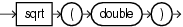Purpose

sqrt returns the correctly rounded positive square root of a double value as a double.

Examples

Consider the query q64 in Example 9-85. Given the data stream SFunc with schema (c1 integer, c2 float, c3 bigint) in Example 9-86, the query returns the stream in Example 9-87.

Example 9-85 sqrt Function Query

<query id="q64"><![CDATA[
select sqrt(c2) from SFunc
]]></query>

Example 9-86 sqrt Function Stream Input

Timestamp   Tuple
10        1,0.5,8
1000        4,0.7,6
1200        3,0.89,12
2000        8,0.4,4

Example 9-87 sqrt Function Stream Output

Timestamp   Tuple Kind  Tuple
10:       +           0.70710677
1000:       +           0.83666
1200:       +           0.9433981
2000:       +           0.6324555

tan

Syntax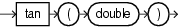Purpose

tan returns the trigonometric tangent of a double angle as a double.

Examples

Consider the query q62 in Example 9-88. Given the data stream SFunc with schema (c1 integer, c2 double, c3 bigint) in Example 9-89, the query returns the stream in Example 9-90.

Example 9-88 tan Function Query

<query id="q62"><![CDATA[
select tan(c2) from SFunc
]]></query>

Example 9-89 tan Function Stream Input

Timestamp   Tuple
10        1,0.5,8
1000        4,0.7,6
1200        3,0.89,12
2000        8,0.4,4

Example 9-90 tan Function Stream Output

Timestamp   Tuple Kind  Tuple
10:       +           0.5463025
1000:       +           0.8422884
1200:       +           1.2345995
2000:       +           0.42279324

tanh

Syntax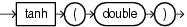Purpose

tanh returns the hyperbolic tangent of a double value as a double.

Examples

Consider the query q90 in Example 9-91. Given the data stream SFunc with schema (c1 integer, c2 double, c3 bigint) in Example 9-92, the query returns the stream in Example 9-93.

Example 9-91 tanh Function Query

<query id="q90"><![CDATA[
select tanh(c2) from SFunc
]]></query>

Example 9-92 tanh Function Stream Input

Timestamp   Tuple
10        1,0.5,8
1000        4,0.7,6
1200        3,0.89,12
2000        8,0.4,4

Example 9-93 tanh Function Stream Output

Timestamp   Tuple Kind  Tuple
10:       +           0.46211717
1000:       +           0.6043678
1200:       +           0.7113937
2000:       +           0.37994897

todegrees

SyntaxPurpose

todegrees converts a double angle measured in radians to an approximately equivalent angle measured in degrees as a double.

The conversion from radians to degrees is generally inexact; do not expect COS(TORADIANS(90.0)) to exactly equal 0.0.

Examples

Consider the query q91 in Example 9-94. Given the data stream SFunc with schema (c1 integer, c2 double, c3 bigint) in Example 9-95, the query returns the stream in Example 9-96.

Example 9-94 todegrees Function Query

<query id="q91"><![CDATA[
select todegrees(c2) from SFunc
]]></query>

Example 9-95 todegrees Function Stream Input

Timestamp   Tuple
10        1,0.5,8
1000        4,0.7,6
1200        3,0.89,12
2000        8,0.4,4

Example 9-96 todegrees Function Stream Output

Timestamp   Tuple Kind  Tuple
10:       +           28.64789
1000:       +           40.107044
1200:       +           50.993244
2000:       +           22.918312

Syntax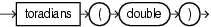Purpose

toradians converts a double angle measured in degrees to an approximately equivalent angle measured in radians as a double.

Examples

Consider the query q92 in Example 9-97. Given the data stream SFunc with schema (c1 integer, c2 double, c3 bigint) in Example 9-98, the query returns the stream in Example 9-99.

<query id="q92"><![CDATA[
]]></query>

Example 9-98 toradians Function Stream Input

Timestamp   Tuple
10        1,0.5,8
1000        4,0.7,6
1200        3,0.89,12
2000        8,0.4,4

Example 9-99 toradians Function Stream Output

Timestamp   Tuple Kind  Tuple
10:       +           0.008726646
1000:       +           0.012217305
1200:       +           0.0155334305
2000:       +           0.006981317

ulp

Syntax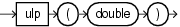Purpose

ulp returns the size of an ulp of the double argument as a double. In this case, an ulp of the argument value is the positive distance between this floating-point value and the double value next larger in magnitude.

Examples

Consider the query q93 in Example 9-100. Given the data stream SFunc with schema (c1 integer, c2 double, c3 bigint) in Example 9-101, the query returns the stream in Example 9-102.

Example 9-100 ulp Function Query

<query id="q93"><![CDATA[
select ulp(c2) from SFunc
]]></query>

Example 9-101 ulp Function Stream Input

Timestamp   Tuple
10        1,0.5,8
1000        4,0.7,6
1200        3,0.89,12
2000        8,0.4,4

Example 9-102 ulp Function Stream Output

Timestamp   Tuple Kind  Tuple
10:       +           1.110223E-16
1000:       +           1.110223E-16
1200:       +           1.110223E-16
2000:       +           5.551115E-17

ulp1

Syntax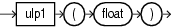Purpose

ulp1 returns the size of an ulp of the float argument as a float. An ulp of a float value is the positive distance between this floating-point value and the float value next larger in magnitude.

Examples

Consider the query q94 in Example 9-103. Given the data stream SFunc with schema (c1 integer, c2 double, c3 bigint) in Example 9-104, the query returns the relation in Example 9-105.

Example 9-103 ulp1 Function Query

<query id="q94"><![CDATA[
select ulp1(c2) from SFunc
]]></query>

Example 9-104 ulp1 Function Stream Input

Timestamp   Tuple
10        1,0.5,8
1000        4,0.7,6
1200        3,0.89,12
2000        8,0.4,4

Example 9-105 ulp1 Function Relation Output

Timestamp   Tuple Kind  Tuple
10:       +           5.9604645E-8
1000:       +           5.9604645E-8
1200:       +           5.9604645E-8
2000:       +           2.9802322E-8